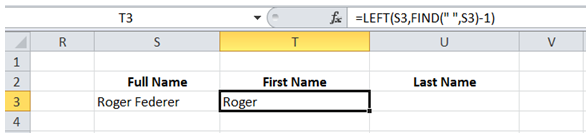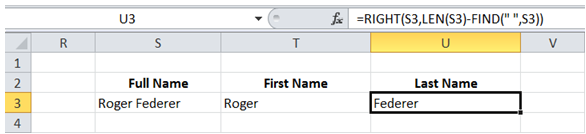## Extract first last name in Excel

For example, I want to separate the First Name and Last Name from the cell value "Roger Federer" and have them in separate cells.

To do it in Excel, here is the answer:

a) Enter the formula =LEFT(S3,FIND(" ",S3)-1) in the cell where the "First Name" is required.

The formula "FIND(" ",S3)" returns the position of the space character. Subtracting 1 returns the position of last character of First Name.

The LEFT function is then used to extract all the characters upto last character of First Name.b) Enter the formula =RIGHT(S3,Len(S3)-FIND(" ",S3)) in the cell where the "Last Name" is required.

The formula "FIND(" ",S3)" returns the position of the space character. Last Name is to the right of this space character. Subtracting the position of space character from length of original string gives the length of Last Name.

The RIGHT function is then used to extract all the characters after space character.